# Gödel interpretation

(diff) ← Older revision | Latest revision (diff) | Newer revision → (diff)

of intuitionistic arithmetic

A translation of formulas of intuitionistic arithmetic into formulas of the type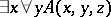, where,andare variables of various finite types. Provable formulas of arithmetic are translated into provable formulas of a quantifier-free theory of finite types. Thus, this translation reduces the consistency of intuitionistic arithmetic (and hence of classical arithmetic) to that of such a theory of finite types, as was Gödel's original aim.

This type theory, call it, has an infinite list of variables of each type: 1) a type(the type of natural numbers); and 2) if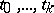are types, then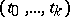is a type (the type of functions which takearguments of types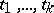, respectively, into a value of type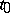). The languagecontains terms of various types: a variable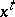of typeis a term of type, 0 is a term of type 0, and the symbolwhich denotes the function of adding one to a natural number is a term of type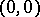. The remaining terms are formed by generation laws: Church-abstraction and primitive recursion for functions of arbitrary type. The atomic formulas ofare equalities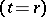, where,are terms of type zero. The formulas ofare obtained from atomic formulas with the aid of the logical connectives of propositional calculus: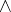,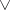,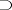,. The postulates ofare the axioms and derivation rules of intuitionistic propositional calculus, equality axioms, the Peano axioms for 0 and, equations of primitive recursion, the axiom of application of a function defined by-abstraction, and, finally, the principle of mathematical induction, formulated as a derivation rule without the use of quantifiers.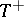will denote the theorycompleted by quantifiers in variables of arbitrary type and corresponding logical axioms and derivation rules for quantifiers.

Gödel's interpretation translates any formulafrom(i.e. any formula of intuitionistic arithmetic as well) into a formula of the type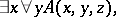where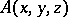is a quantifier-free formula,,,are variables of different types, andis the set of all free variables of the formula.

Letbe a formula of intuitionistic arithmetic, and letbe its Gödel interpretation. Ifis deducible in formal intuitionistic arithmetic, it is possible to construct a term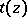ofsuch that the formula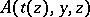is deducible in. Thus, the consistency of arithmetic is reduced to demonstrating the consistency of the quantifier-free theory.

The intuitionistic semantics based on Gödel's interpretation is defined as follows: A formulais considered to be true if it is possible to find a computable termsuch that the quantifier-free formulais true for any computable.

How to Cite This Entry:
Gödel interpretation. Encyclopedia of Mathematics. URL: http://encyclopediaofmath.org/index.php?title=G%C3%B6del_interpretation&oldid=15859
This article was adapted from an original article by A.G. Dragalin (originator), which appeared in Encyclopedia of Mathematics - ISBN 1402006098. See original article